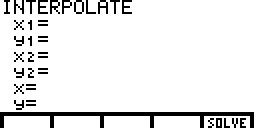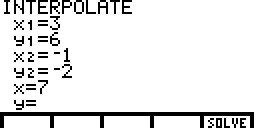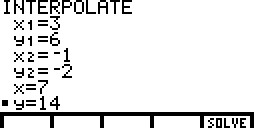# Knowledge Base

## Solution 10449: Using the Interpolate Editor on the TI-85 and TI-86.

### How do I use the interpolate editor on the TI-85 or TI-86?

The following instructions demonstrate how to use the interpolate/extrapolate feature of the TI-85 and TI-86.

Example: Given points (3,6) and (-1,-2), find the y value at x=7.

• Press [2nd] [MATH] [MORE] [F1] to enter the editor.• At x1=, input 3 and press [ENTER].

• At y1=, input 6 and press [ENTER].

• At x2=, input -1 and press [ENTER].

• At y2=, input -2 and press [ENTER].

• At x=, input 7 and press [ENTER].• At y=, press [F5] to solve for the y value. The calculator should display 14 as the solution.